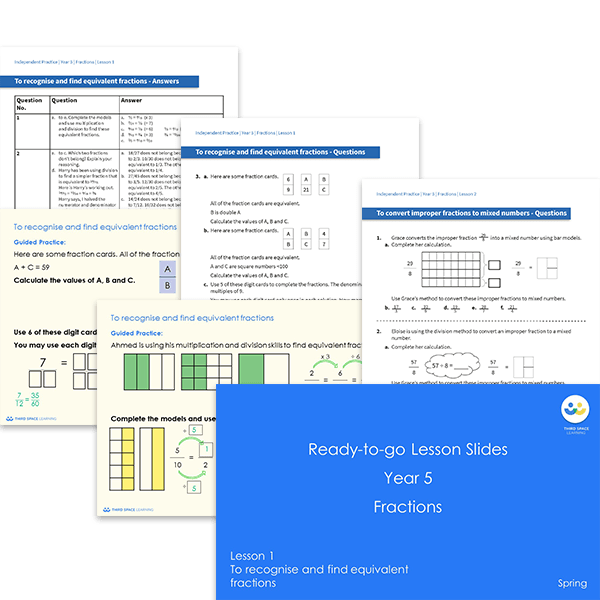# Ready-to-go lessons Year 5 Fractions (Spring Block 2) Slides and Worksheets

Fractions ready-to-go lessons for Year 5 includes 20 PowerPoints with matching worksheets ready for you to use with a class. These resources follow a mastery approach to mathematics teaching, similar to schemes such as White Rose Maths and Mathematics Mastery. The PowerPoints are fully editable with detailed notes for every teaching slide. Each associated worksheet is divided into three parts with a range of activities to complete across the block.

This Year 5 Fractions pack contains 20 fully editable PowerPoints and 20 worksheets.

The slides and worksheets cover:

• Recognising and finding equivalent fractions
• Converting improper fractions to mixed numbers and converting mixed numbers to improper fractions
• Counting forwards and backwards in fractions
• Comparing and ordering fractions less than one and greater than one
• Adding fractions (including with the same denominator, within 1, adding three or more fractions, where the total is greater than one and mixed numbers)
• Subtracting fractions (including with the same denominator, breaking the whole, mixed numbers)
• Multiplying a unit fraction, non-unit fraction and mixed number by an integer
• Finding a fraction of an amount and using fractions as operators

Year 5

Fractions